# Maps in R

I have spent quite a lot of time lately learning to use the R programming language for my work. Mainly I use it to perform statistical analyses, and I have to say that I am quickly falling in love with it. I had limited experience using Stata during grad school, and I never really liked it very much. But I have learned some Python and even C++ in the past, and since R is a full programming language its use is much more familiar and intuitive to me.

Moreover, R is powerful, and its use is not limited to statistics. I have been using the ggplot2 package to draw plots for my research, and that led me to try to see if I could draw maps with it. It turns out it can, and it is in fact quite easy and powerful. So in this blog post I basically paste a tutorial that I have been putting together. It is quite long. The goal of the tutorial is to draw a world map in which the countries are colored according to the percentage of their population that has access to an improved source of water. I only do that at the very end of the tutorial, but before I have, in quite a lot of detail, tried to document the process through which I learned to put it together. I start with what you need to do to draw a basic world map, and then I move on to projections, drawing country borders and cities, and finally the download of data and the final plot. The tutorial also discusses some basic data manipulation. R is very powerful for that task, and the use of the dplyr package really makes it a breeze.

In order to learn to draw maps myself, I started from a tutorial that you can find here: http://rpsychologist.com/working-with-shapefiles-projections-and-world-maps-in-ggplot

What I do here is just an extension and more in-depth explanation of what the author did. The first part of this post, then, follows that tutorial quite closely, and only later on I do things that are not included there. The big difference, however, is that I really try to explain everything that I did. This is mostly the process through which I learned the commands myself, so you will have to forgive me if at some point the phrasing is not too clear. I have not edited this for style or comprehension, so please drop me a line if something is not clear or there is anything I could improve.

Also, I am by no means an expert. Some of the things I write here are the way I figured out the commands work, but I could definitely be wrong. Feel free to let me know if that is the case.

The assumption here is that you are familiar with basic R and to plotting with ggplot2. If you are not, you might want to start somewhere else (there are many resources online to learn R) and come back afterwards. Anyways, without further ado, here is the tutorial.

``````setwd("~/Documents/Research/R/Maps")
unzip("ne_110m_land.zip")
unzip("ne_110m_populated_places.zip")
``````

It is now time to load the libraries that we’ll need. If these are not installed yet, you need to do that with `install.packages("package.name")`

``````library(rgdal)
library(ggplot2)
library(plyr)
library(dplyr)
library(scales)
``````

In some of the steps below, you might get an error term about `gpclibPermitStatus` being FALSE (you might not, in which case you can skip this step). You can take care of that by running `gpclibPermit()` (you might need to install the maptools package first)

``````library(maptools)
gpclibPermit()
``````

Now that we have our tools, we start by reading the map and putting it into a SpatialPolygonsDataFrame. We do that with the `readOGR()` function from the rgdal package. The first parameter (dsn) is the folder where the map data is located. Since we are already in that folder (we set it up as the working directory where we unzipped all the files we downloaded), we indicate it with a single dot: “.”. The second parameter is the layer (the map) that we want to load. The shapefiles for a given map all have the same name with different extensions (.shp, .shx, .dbf, .prj). Here we indicate just the name without any extension.

``````wmap <- readOGR(dsn=".", layer="ne_110m_land")
``````
``````## OGR data source with driver: ESRI Shapefile
## Source: ".", layer: "ne_110m_land"
## with 127 features and 2 fields
## Feature type: wkbPolygon with 2 dimensions
``````

At this point, wmap contains all the info of the map. However, since we are planning on drawing the map with ggplot, we first need to convert it to a data frame. We do that with the `fortify()` function

``````wmap_df <- fortify(wmap)
``````
``````## Regions defined for each Polygons
``````

In order to prepare our plot, we now build a blank theme for ggplot

``````theme_opts <- list(theme(panel.grid.minor = element_blank(),
panel.grid.major = element_blank(),
panel.background = element_blank(),
plot.background = element_rect(fill="white"),
panel.border = element_blank(),
axis.line = element_blank(),
axis.text.x = element_blank(),
axis.text.y = element_blank(),
axis.ticks = element_blank(),
axis.title.x = element_blank(),
axis.title.y = element_blank(),
plot.title = element_text(size=22)))
``````

Now we can draw the map. Notice that we draw the polygons in the map with `geom_polygon()`, and that besides the x and y of each point of the polygons (the converted long and lat), we also determine the groupings using the group variable of the map data frame (there will always be one in the shapefile for each polygon). We add `coord_equal()`, which sets the ratio between the x and y scales. The default ratio is 1, which means that adding this to the plot will make sure that it does not get flattened or narrowed

``````ggplot(wmap_df, aes(long, lat, group=group)) +
geom_polygon() +
coord_equal() +
theme_opts
``````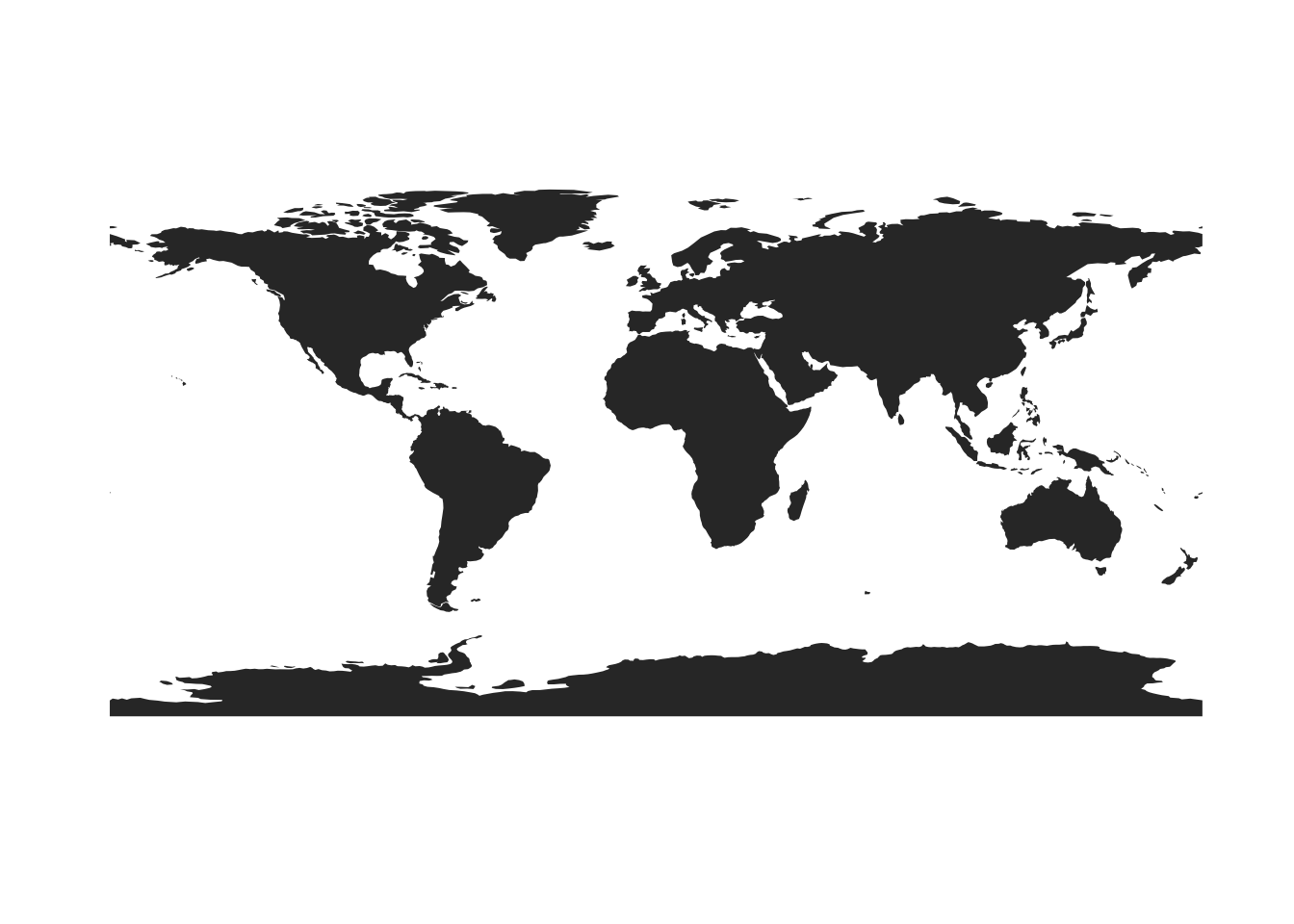This map, however, has merely plotted the longitude and latitude onto a flat surface. Since the world is spherical, this makes the map look weird. The further north and south we go, the more stretched out horizontally the image looks. There is not one single way of projecting longitude and latitude onto a flat surface, but we can transform our original data using a number of ‘projections’. Here, we use the spTransform() function to project our world map using the Robinson projection. The function is self-explanatory: you first pass it your map object, and then indicate the Coordinate Reference System (CRS) to which you want to project it. There are different ways of defining and referring to a CRS, and the +proj in the function is one way of doing so.

``````wmap_robin <- spTransform(wmap, CRS("+proj=robin"))
``````

Like before, once we have our map projected onto a Robinson coordinate reference system, we can make it into a data frame with `fortify()`.

``````wmap_robin_df <- fortify(wmap_robin)
``````
``````## Regions defined for each Polygons
``````

And then we draw it with `ggplot()`

``````ggplot(wmap_robin_df) +
geom_polygon(aes(long, lat, group=group)) +
coord_equal() +
theme_opts
``````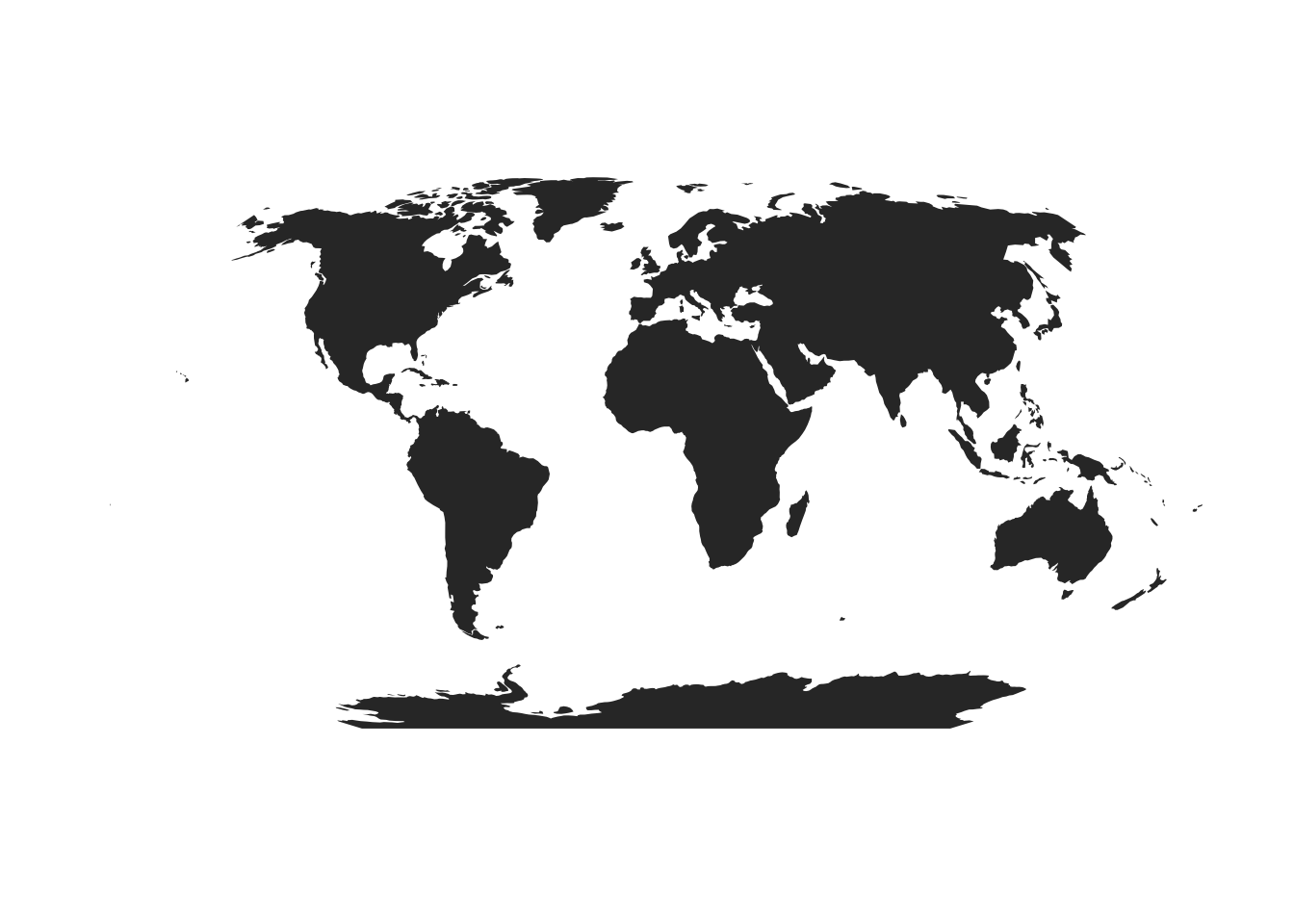As you can see, the map now seems to be bending to embrace the spheric shape of the earth, and the large latitudes seem less distorted than before.

Now the problem here is that, in a map, there are two types of polygons. Those that delimit land and those that delimit ‘holes’ in the land (for instance lakes). Unless we tell ggplot to fill these ‘holes’ differently (as water instead of land), they will all be filled as land and therefore we will not be able to see the lakes. In this map, the only visible such case is the Caspian sea. In order to fix this, we tell ggplot to ‘fill’ the holes based on the variable ‘hole’ (it has this name in the map file, and has TRUE and FALSE values), thus the new `fill=hole` component in `geom_polygon()`

``````ggplot(wmap_robin_df) +
geom_polygon(aes(long, lat, group=group, fill=hole)) +
coord_equal() +
theme_opts
``````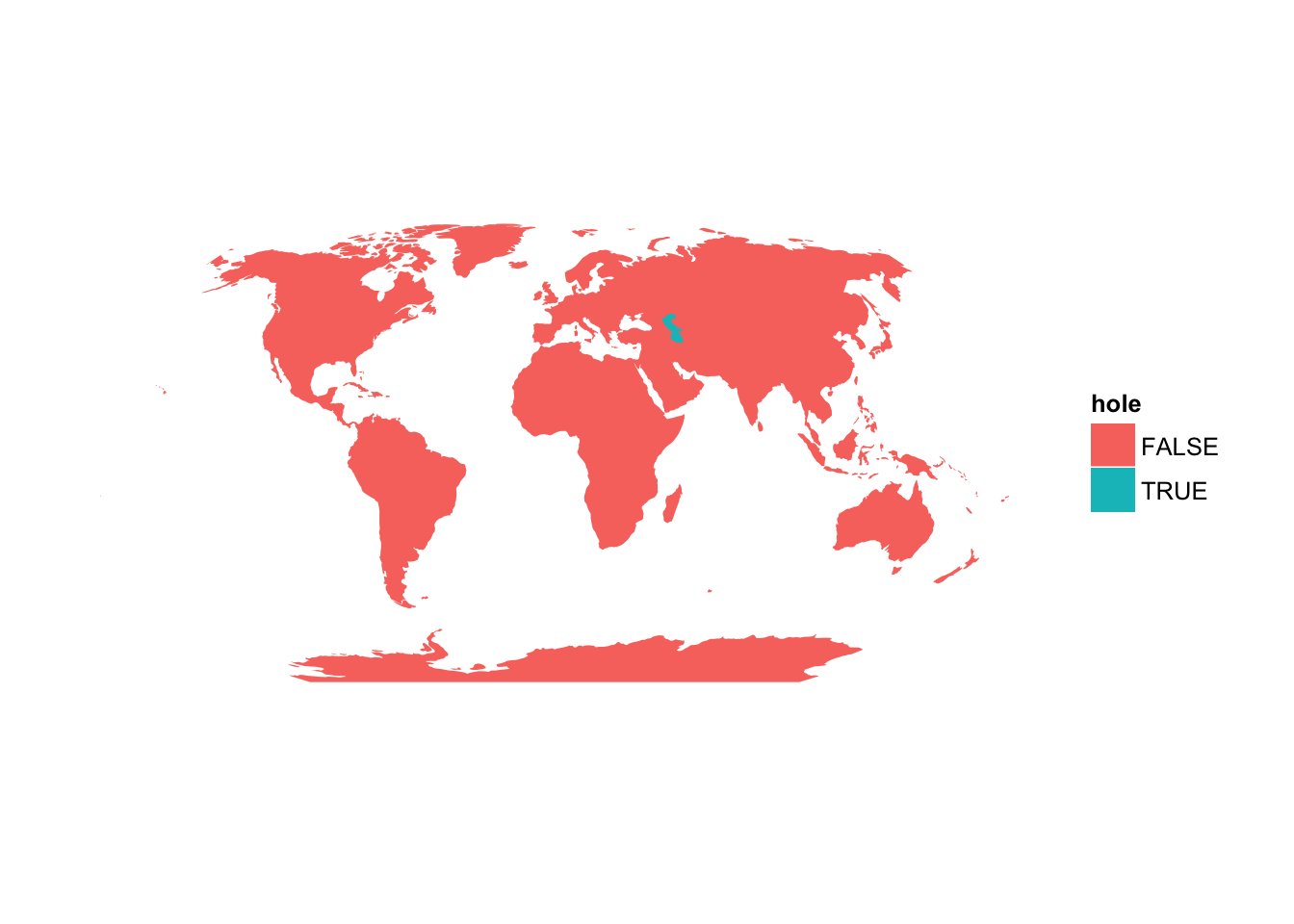But the moment we do this, ggplot automatically does the same that it would do if we asked it to fill different elements according to the values of a variable: it gives us two very different colors and inserts a legend box to tell us about them. The good news is that we can definitely see the Caspian sea. But in order to make the colors more palatable and remove the useless legend, we need to add a few more elements to ggplot. We introduce `scale_fill_manual()` in order to be able to establish ourselves the features of the ‘fill’. The ‘values’ are the colors, of which we need two (one for each value of the ‘hole’ variable, true and false), so we say that one of the values will be ‘black’ and the other will be ‘white’, just as the oceans are painted now, which will give the Caspian sea the same color. We also say that we do not want the legend by adding `guide="none"`

``````ggplot(wmap_robin_df) +
geom_polygon(aes(long, lat, group=group, fill=hole)) +
coord_equal() +
theme_opts +
scale_fill_manual(values=c("black", "white"), guide="none")
``````This map looks exactly like the one we had before we introduced the ‘fill’ element, but now we can see the Caspian sea.

This is pretty and such, but we also want to make the map useful. We will try to draw the countries of the world. For that, we need to load the map with the polygons of the countries. The process is exactly as we did above

``````wmap_countries <- readOGR(dsn=".", layer="ne_110m_admin_0_countries")
``````
``````## OGR data source with driver: ESRI Shapefile
## with 177 features and 63 fields
## Feature type: wkbPolygon with 2 dimensions
``````

Now we project the map to the same system of coordinates, Robinson

``````wmap_countries_robin <- spTransform(wmap_countries, CRS("+proj=robin"))
``````

And turn it into a data frame that we can draw with ggplot with `fortify()`

``````wmap_countries_robin_df <- fortify(wmap_countries_robin)
``````
``````## Regions defined for each Polygons
``````

Now we are ready to draw the map. However, notice that for the countries we have no interest in drawing the whole polygon, but only the borders. We do that by using `geom_path()` instead of `geom_polygon()`. We indicate that we want the borders to appear in white, and with a line of size 0.3. Everything else is exactly as before

``````ggplot(wmap_robin_df) +
geom_polygon(aes(long, lat, group=group, fill=hole)) +
geom_path(data=wmap_countries_robin_df, aes(long, lat, group=group), color="white", size=0.3) +
coord_equal() +
theme_opts +
scale_fill_manual(values=c("black", "white"), guide="none")
``````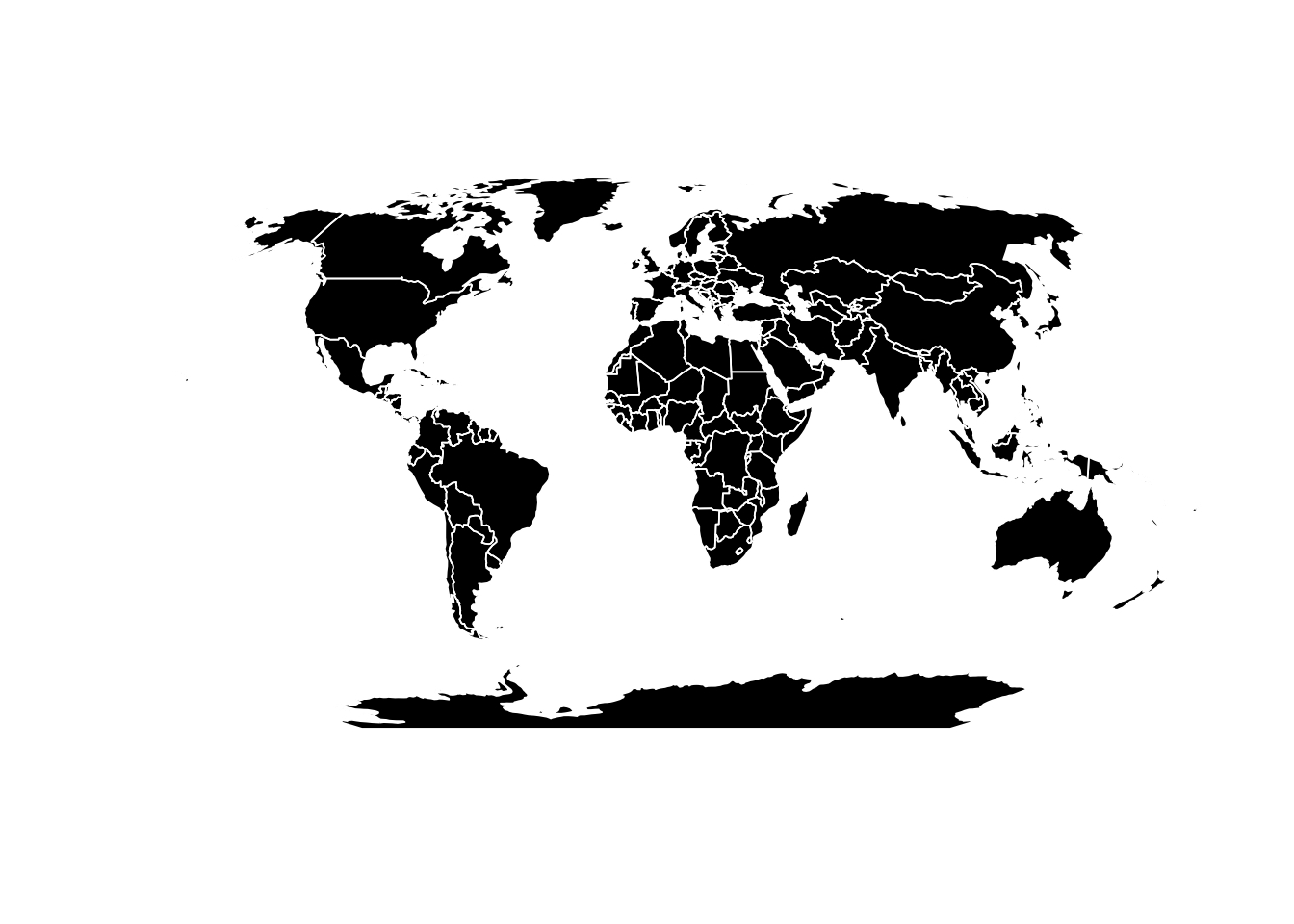Let’s now make the map a bit more interesting by adding info about cities within the map. In order to do that, let’s load the corresponding data.

``````places <- readOGR(dsn=".", layer="ne_110m_populated_places")
``````
``````## OGR data source with driver: ESRI Shapefile
## Source: ".", layer: "ne_110m_populated_places"
## with 243 features and 92 fields
## Feature type: wkbPoint with 2 dimensions
``````

Same as with the other files, we will transform it to a Robinson projection

``````places_robin <- spTransform(places, CRS("+proj=robin"))
``````

ASIDE

Ok, none of what I do here is necessary to understand how the map is drawn, so feel free to skip to the end of this aside further down. I just want to provide some information about the files that we are working with. These are not data frames, which is precisely why we convert them into data frames with `fortify()` so that we can draw them with ggplot. The types of objects that we are using contain the basic information to draw the map, but they also have a data frame in them with basic information about each polygon, or point, or whatever its components are. In the country file, the data frame contains the codes and names of the countries, etc., and in this file for cities, it contains the names of the cities, their population for different years, etc. We can access this data frame inside the spatial objects by referring to it as @data. We can see the first few elements of this data dataframe by doing this (I omit the output here because it is rather long):

``````head(places_robin@data)
``````

If you run `class(places@data)` you will see that this is a data frame. Then you can access each of the columns in the exact same way you would for any other data frame:

``````head(places_robin@data\$POP2000)
``````
``````##  0 0 0 0 0 0
``````

In fact, you can even do this and it will work equally well:

``````head(places_robin\$POP2000)
``````
``````##  0 0 0 0 0 0
``````

The info that we will want to use to plot the cities, the population in the year 2000, then, is in the @data part of the object

END OF ASIDE

If we followed the same porcess as before, we would `fortify()` our spatial data to turn it into a data frame and be able to draw it with ggplot. But look what happens if we try:

``````places_robin_df <- fortify(places_robin)
``````
``````## Error: ggplot2 doesn't know how to deal with data of class SpatialPointsDataFrame
``````

We are told that ggplot cannot deal with data of class SpatialPointsDataFrame. Notice that this is not the same kind of object that we had before for the world and countries maps, which were of class SpatialPolygonsDataFrame (you can check by running `class()` on the different objects). In the objects containing the previous maps, we had polygons, whereas here we only have points. So what are we going to do if we can’t use `fortify()`? Luckily, things are much easier now. Since we only have points, not polygons, the SpatialPointsDataFrame can be turned into a data frame almost directly without the need of complex transformations, and thus this task can be handled by basic R commands. So we do:

``````places_robin_df <- as(places_robin, "data.frame")
``````

Alternatively, we could do:

``````places_robin_df <- as.data.frame(places_robin)
``````

Both expressions do exactly the same. You can check by trying both and (if you give them different names) compare them with either `identical(places_robin_df1, places_robin_df2)` or with `all.equal(places_robin_df1, places_robin_df2)`. `identical()` only tells you if the two objects are the same (TRUE or FALSE), whereas `all.equal()` will tell you what the differences are if there are any.

If we used `as.data.frame()` with one of the SpatialPolygonsDataFrame objects containing the previous maps we would also get a data frame, but it would only contain the @data part of the object, not the coordinates of the polygons, whereas in the case of SpatialPointsDataFrame objects the point coordinates are attached to the end of the resulting data frame. That’s why in the former case we had to use `fortify()`.

`places_robin_df` is then a data frame that contains everything we need, the coordinates of the points where the cities are located in the Robinson projection, as well as all the @data stuff, which has the population variables (as well as names of cities and other variables).

The coordinates that determine the location for each city from `places_robin` are now at the very end of the data frame in two columns labeled “coords.x1” and “coords.x2”. The data frame contains also columns with names LONGITUDE and LATITUDE, but those are NOT the ones we want. These existed already in the @data part of places before, and they also contained the coordinates of the cities, but because they were in the @data part they were not transformed to the Robinson projection when we used `spTransform()`, and trying to use them will not give us what we want. We then rename the two last columns with the coordinates that we need so that they reflect that they are the longitude and latitude of the cities in the dataset. We see from running `names(places_robin_df)` that the two variables are the 93rd and 94th in the data set, so we use their index to change their names:

``````names(places_robin_df) <- "long"
names(places_robin_df) <- "lat"
``````

Now we can draw the map. Notice a few things more that we’ve done here. The data from the cities is added with `geom_point()`, because they are points in the map (duh). Thus we do not use `group` or `fill`, and they are set to NULL. We set the size of the points to reflect POP2000 (one of the variables originally contained in the @data portion of the object), that is, the population size of the city in the year 2000. We give them a blue color, and a transparency with the `alpha` parameter (which has to be a value between 0 and 1, here expressed as a fraction, and the `I` indicates that it is a set aesthetic). We have also added a `scale_size_continuous()` to determine the range of size of the points. Since we set it between 1 and 20, the city with the smallest population will have a point of size 1, the city with the largest population will have a point size of 20, and all the values in between will have the proportionate size on that range. We also indicate that we do not want the legend for the sizes to be displayed with `guide="none"`

``````ggplot() +
geom_polygon(data=wmap_countries_robin_df, aes(long,lat, group=group, fill=hole)) +
geom_point(data=places_robin_df, aes(long, lat, group=NULL, fill=NULL, size=POP2000), color="#32caf6", alpha=I(8/10)) +
geom_path(data=wmap_countries_robin_df, aes(long,lat, group=group, fill=hole), color="white", size=0.3) +
coord_equal() +
theme_opts +
scale_fill_manual(values=c("black", "white"), guide="none")+
scale_size_continuous(range=c(1,20), guide="none")
``````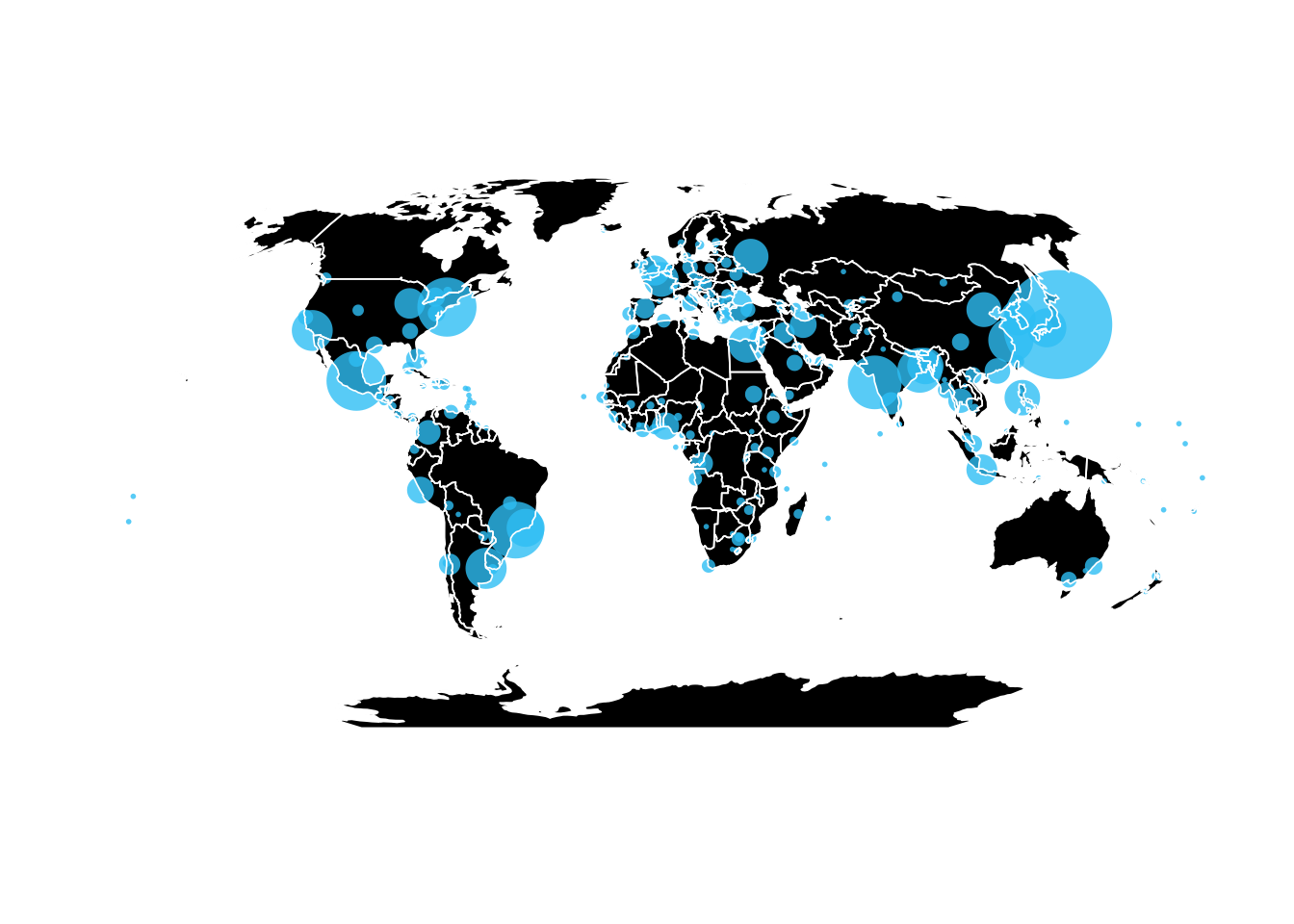Now we are going to try to color the countries. As a first, completely useless step, we will just give each country a different color, without these meaning anything in particular. In order to do so, we only need to modify our map with countries slightly (for this exercise, we will remove the points with the data for the cities):

``````ggplot(wmap_countries_robin_df) +
geom_polygon(aes(long, lat, group=group, fill=id)) +
coord_equal() +
theme_opts +
scale_fill_manual(values=1:177, guide="none")
``````